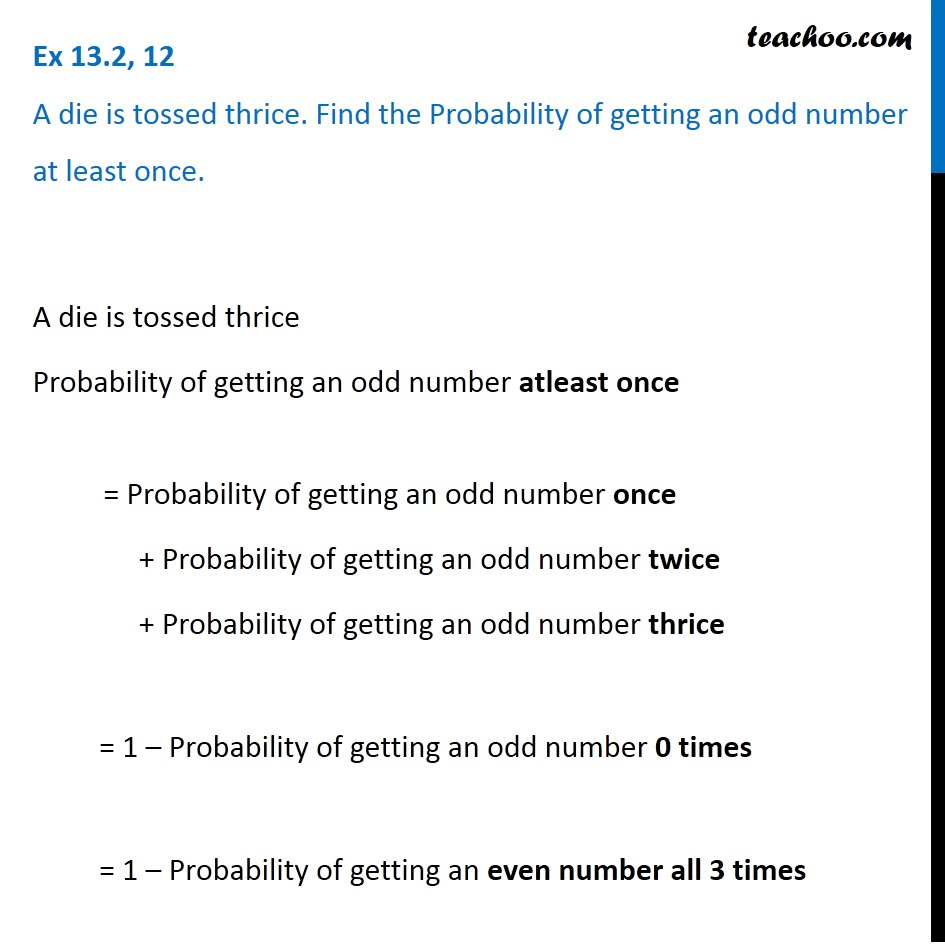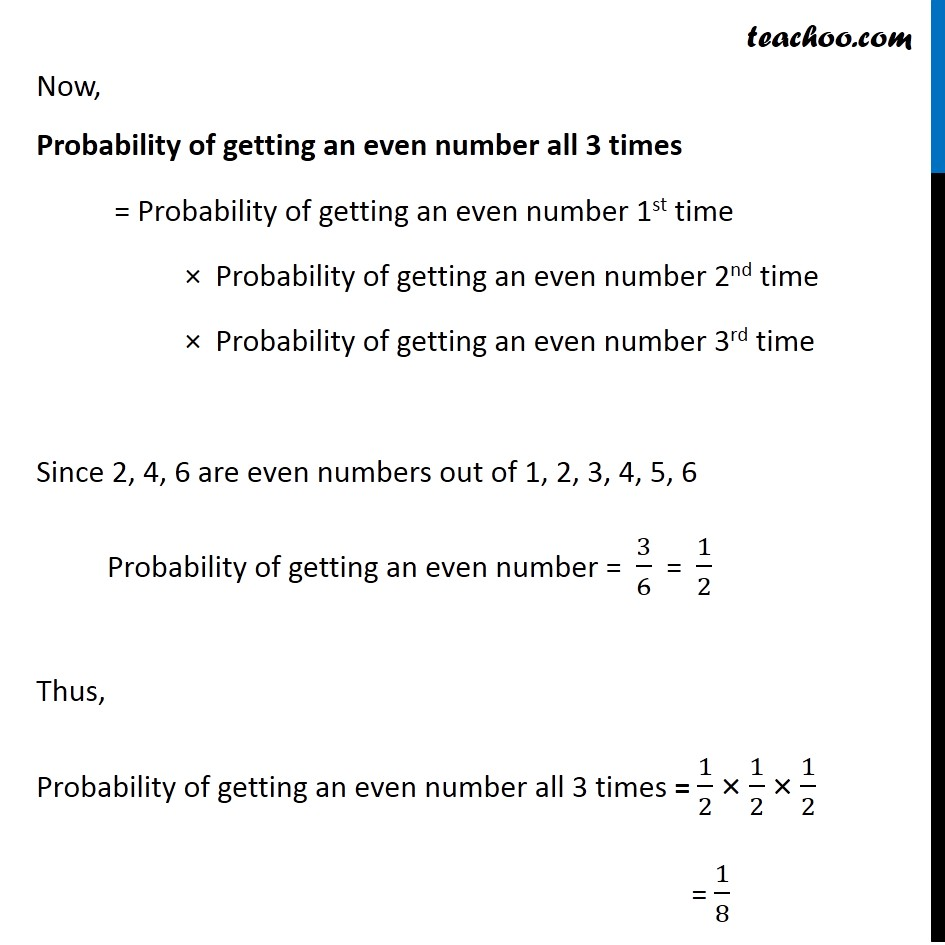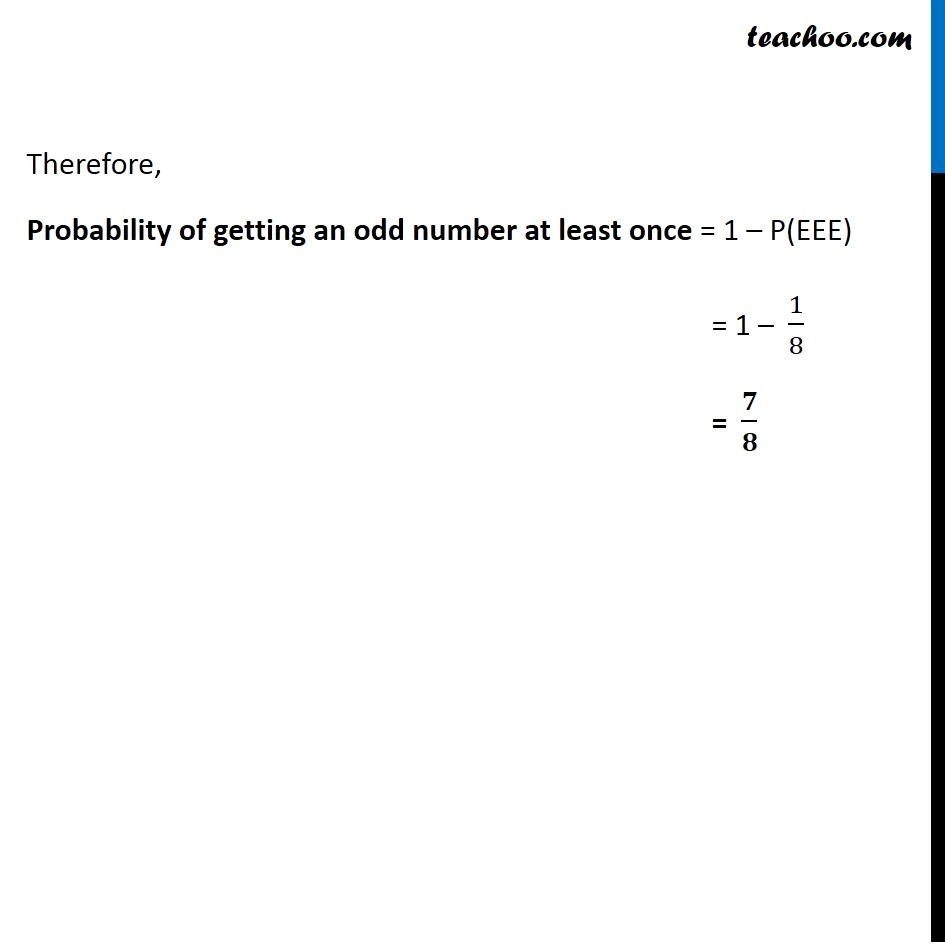Ex 13.2

Chapter 13 Class 12 Probability
Serial order wiseLearn in your speed, with individual attention - Teachoo Maths 1-on-1 Class

### Transcript

Ex 13.2, 12 A die is tossed thrice. Find the Probability of getting an odd number at least once.A die is tossed thrice Probability of getting an odd number atleast once = Probability of getting an odd number once + Probability of getting an odd number twice + Probability of getting an odd number thrice = 1 – Probability of getting an odd number 0 times = 1 – Probability of getting an even number all 3 times = 1 − 1/2 × 1/2 × 1/2 = 1 − 1/8 = 𝟕/𝟖